GCSE Maths Geometry and Measure 3D Shapes Sphere

Surface Area Of A Sphere

# Surface Area Of A Sphere

Here we will learn about the surface area of a sphere, including how to calculate the surface area of a sphere given its radius and the surface area of a hemisphere.  We will look at some practice problems.

There are also surface area of a sphere worksheets based on Edexcel, AQA and OCR exam questions, along with further guidance on where to go next if you’re still stuck.

## What is the surface area of a sphere?

The surface area of a sphere is the area which covers the outer surface of a sphere.

The formula for the surface area of a sphere with radius r is:

\text{Surface area}=4\pi{r}^{2}

Notice that the square of the radius (r^2) occurs within the surface area formula.

The surface area of a shape uses two-dimensions so the units for surface area are units squared.

### What is the surface area of a sphere?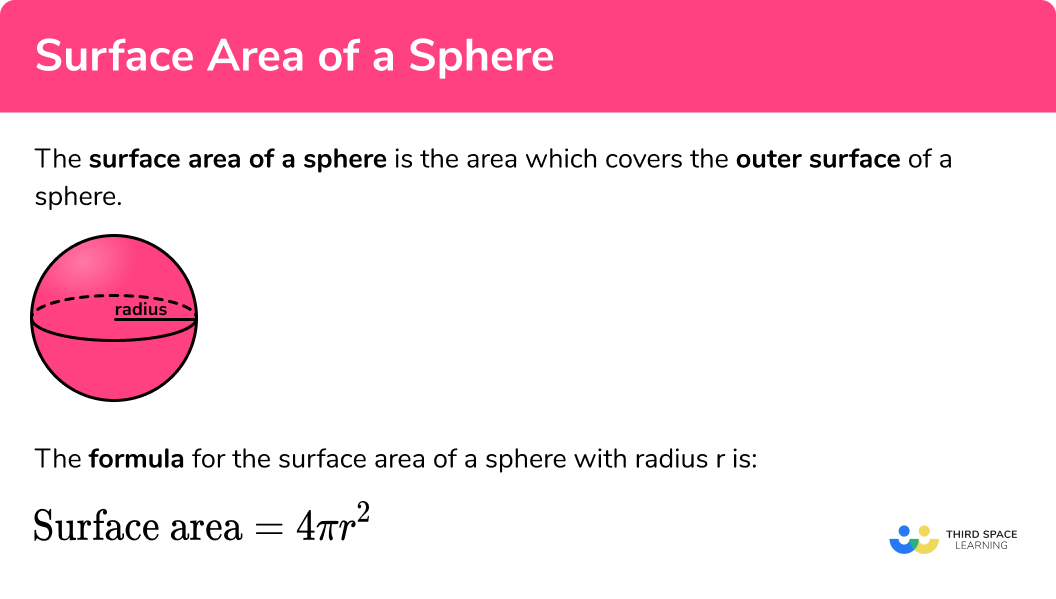## How to calculate the surface area of a sphere

In order to calculate the surface area of a sphere:

1. Write down the formula.
2. Substitute the given values into the formula.
3. Complete the calculation.
4. Write the final answer, including the units.

### How to calculate the surface area of a sphere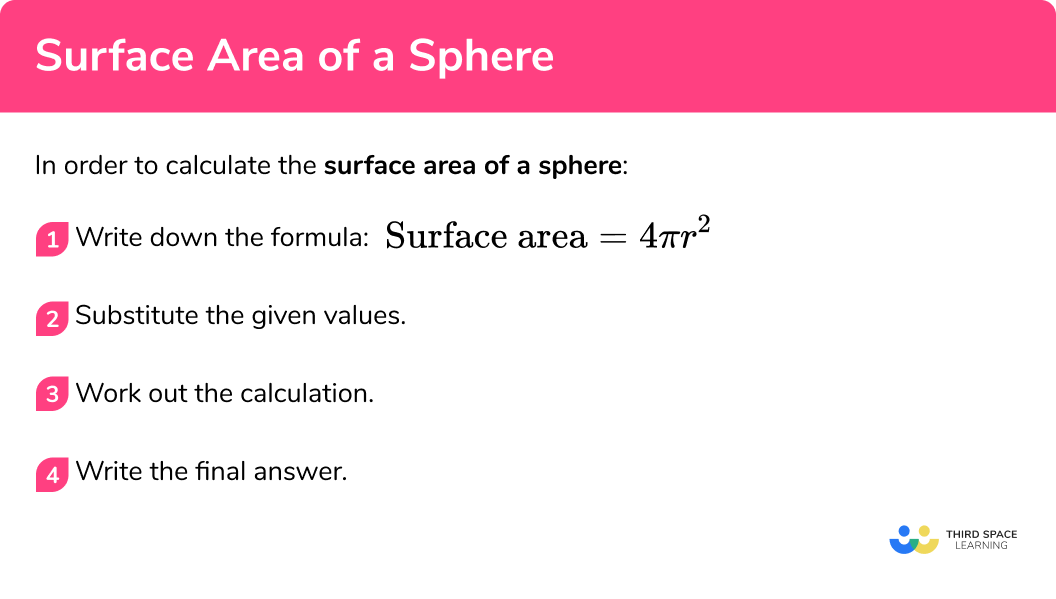### Related lessons on sphere

Surface area of a sphere is part of our series of lessons to support revision on sphere. You may find it helpful to start with the main sphere lesson for a summary of what to expect, or use the step by step guides below for further detail on individual topics. Other lessons in this series include:

## Surface area of a sphere examples

### Example 1: surface area of a sphere given the radius

Find the surface area of the sphere below. Write your answer to 1 decimal place.

1. Write down the formula.

To answer the question we need to use the formula for the surface area of a sphere:

\text{Surface area}=4 \pi r^2

2Substitute the given values into the formula.

The radius (the distance from the centre of the sphere to the surface) is 4.8 \; cm. We need to substitute the value of the radius into the formula for the surface area of a sphere:

\text{SA }=4 \times \pi \times 4.8^2

3Complete the calculation.

Use a calculator to work out the value for the surface area:

\begin{aligned} \text{SA }&=4 \times \pi \times 4.8^2\\\\ &=\frac{2304}{25}\pi\\\\ &=289.529179…… \end{aligned}

4Write the final answer, including the units.

Check what form the final answer needs to be. Here we are asked to give the answer to 1 decimal place.

289.529179… = 289.5 \text{ cm}^{2} \ \text{(1dp)}

The surface area of the sphere is 289.5cm^2 \; (1dp).

### Example 2: surface area of a sphere

Find the surface area of a sphere with the diameter of 42 \; mm.

To answer the question we need to use the formula for the surface area of a sphere:

\text{Surface area}=4 \pi r^2

As the diameter is twice the length of the radius, we can divide the diameter by 2 to get the value for r:

\begin{aligned} r&=d\div{2}\\ &=42\div{2}\\ &=21 \end{aligned}

So the radius r=21 \; mm. Now we can substitute r=21 into the formula for the surface area of a sphere to get:

\text{SA }=4 \times \pi \times 21^2

Use a calculator to work out the value.

\begin{aligned} \text{SA }&=4 \times \pi \times 21^2\\\\ &=1764\pi\\\\ &=5541.769441… \end{aligned}

Here we are asked to write the answer to the nearest square millimetre and so:.

5541.769441… = 5542 \ \text{mm}^2 \ \text{(0dp)}

The surface area of the sphere is 5542 \ mm^2 \ (0dp) .

### Example 3: surface area of a sphere – in terms of π

Find the surface area of a sphere with a radius of 6 \; cm.

To answer the question we need to use the formula for the surface area of a sphere:

\text{Surface area}=4 \pi r^2

We need to substitute the value of the radius into the surface area of a sphere formula.

\text{SA }=4 \times \pi \times 6^2

Work out the curved surface area,  focussing on the number parts of the calculation.

\begin{aligned} \text{SA }&=4 \times \pi \times 6^2\\\\ &=144\pi \end{aligned}

Here we are asked to give the answer in terms of \pi

144\pi\text{ cm}^2

The surface area of the sphere is 144 \pi \; cm^2 .

### Surface area of a hemisphere

A hemisphere is half of a sphere although the surface area of a hemisphere is not half of the surface area of a sphere. This is because we have an extra circular face that we have to add onto the surface area of the curved face.

As the surface area of a sphere is equal to 4\pi{r}^{2} , half of this formula would obtain the curved surface area of a hemisphere (the dome). The surface area of the dome is therefore 2\pi{r}^{2} . A circle has an area of \pi{r}^{2} and because we are talking about the same 3 D shape, the radius r is the same. If we add the area of the two surfaces together, we get the surface area of a hemisphere to be:

\begin{aligned} \text{ SA}&=2\pi{r}^{2}+\pi{r}^{2}\\\\ &=3\pi{r}^{2} \end{aligned}

\text{Surface area of a hemisphere }=3\pi{r}^{2}

for a hemisphere with radius r .

## Surface area of a hemisphere examples

### Example 4: surface area of a hemisphere

Calculate the total surface area of a hemisphere with radius 7 \; cm . Write your answer to 1 decimal place.

To answer the question we need to use the formula for the surface area of a hemisphere:

\text{Surface area of a hemisphere}=3 \pi r^2

Substituting r=7 into the formula for the surface area of a hemisphere (SAH) , we get:

SAH=3\times \pi \times 7^2

\begin{aligned} \text{ SAH}&=147\pi\\\\ &=461.8141201... \end{aligned}

Here we are asked to give the answer to 1 decimal place

461.8141201… = 461.8 \ cm^2 \ (1dp)

The surface area of the hemisphere is 461.8 \; cm^2 \; (1dp).

### Example 5: surface area of a hemisphere given the diameter

Calculate the total surface area of a hemisphere with a diameter of 26.4 \; cm . Write your answer in terms of \pi .

To answer the question we need to use the formula for the surface area of a hemisphere:

\text{SAH}=3 \pi r^2

As we area given the diameter, we need to divide this by 2 so that we can find the value of the radius:

\begin{aligned} r&=d\div{2}\\\\ &=26.4\div{2}\\\\ &=13.2 \end{aligned}

Substituting r=13.2 into SAH=3r^2 , we have

\text{SAH }=3\times\pi\times{13.2}^{2}

\begin{aligned} \text{SAH }&=3\times\pi\times{13.2}^2\\\\ &=\frac{13068}{25}\pi \end{aligned}

As we need to leave the answer in terms of \pi , the surface area of the hemisphere is equal to \frac{13068}{25}\pi or 522.75\pi.

The total surface area of the hemisphere is 522.75\pi \; cm^2

### Example 6: radius of a sphere

The surface area of a sphere is 4000 \; cm^2 . Calculate the radius of the sphere. Write your answer to 1 decimal place.

To answer the question we need to use the formula for the surface area of a sphere:

\text{Surface area}=4 \pi r^2

We are given the surface area, so we can substitute this into the formula.

4000=4 \pi r^2

We need to rearrange the formula to find the value of r.

r=17.84124116…=17.8\text{ cm} \; (1dp)

The radius of the sphere is 17.8 \; cm \; (1dp).

### Common misconceptions

• Using the correct formula

There are several formulas that can be used, so we need to match the correct formula to the correct context.

• Rounding

It is important to not round the answer until the end of the calculation. This will mean your final answer is accurate.

• Make sure you have the correct units

For area we use square units such as cm^2 .

For volume we use cube units such as cm^3 .

• Using the radius or the diameter

It is a common error to mix up radius and diameter. Remember the radius is half of the diameter.

• Confusing the volume formula with the surface area formula

It is a common error to mix up the formula for the volume of the sphere with the formula for the surface area of the sphere.

Remember that the surface area formula includes a squared term, whereas the volume formula includes a cubed term.

### Practice surface area of a sphere questions

1. Find the surface area of a sphere of radius 10 \; cm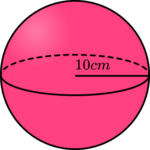314.2 \ cm^2942.5 \ cm^21256.6 \ cm^24188.8 \ cm^2We are finding the surface area of a sphere, so we substitute the value of r into the formula.

\begin{aligned} \text{Surface area}&=4\pi r^2\\\\ &=4 \times \pi \times 10^2\\\\ &=400\pi\\\\ &=1256.637061…\\\\ &=1256.6 \text{ cm}^2 \ (1dp) \end{aligned}

2. Find the surface area of a sphere of diameter 6.4 \; cm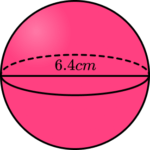32.2 \ cm^2128.7 \ cm^2137.3 \ cm^2514.7 \ cm^2We are finding the surface area of a sphere, so we substitute the value of r into the formula. We have been given the diameter, so we need to halve 6.4 to get the radius, then substitute this value into the formula for the surface area of a sphere.

r=d\div{2}=6.4\div{2}=3.2\text{ cm}.

\begin{aligned} \text{Surface area}&=4\pi r^2\\\\ &=4 \times \pi \times 3.2^2\\\\ &=\frac{1024}{25}\pi\\\\ &=128.679351…\\\\ &=128.7 \text{ cm}^2 \ (1dp) \end{aligned}

3. Calculate the surface area of a sphere of radius 9 \; cm . Leave your answer in terms of \pi .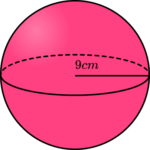324\pi \text{ cm}^236\pi \text{ cm}^281\pi \text{ cm}^2972\pi \text{ cm}^2We are finding the surface area of a sphere, so we substitute the value of r into the formula.

\begin{aligned} \text{Surface area}&=4\pi r^2\\\\ &=4 \times \pi \times 9^2\\\\ &=324\pi\\\\ &=324\pi \text{ cm}^2 \end{aligned}

4. Find the total surface area of a hemisphere with radius 8 \; cm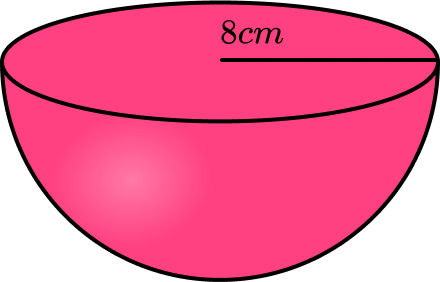201.0 \ cm^2201.1 \ cm^2603.1 \ cm^2603.2 \ cm^2The surface area of a hemisphere is equal to 3\pi{r}^{2} . Substituting r=8 \; cm into the formula, we have

\begin{aligned} \text{SAH }&=3\times\pi\times{8}^2\\\\ &=192\pi\\\\ &=603.1857895…\\\\ &=603.2\text{ cm}^2 \ (1dp) \end{aligned}

5. Find the total surface area of a hemisphere with radius 12 \; cm . Leave your answer in terms of \pi .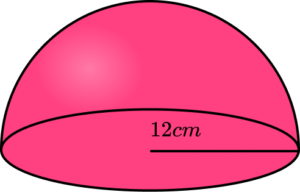432\pi \text{cm}^2144\pi \text{ cm}^2720\pi \text{ cm}^2576\pi \text{ cm}^2The surface area of a hemisphere is equal to 3\pi{r}^{2} . Substituting r=12 \; cm into the formula, we have

\begin{aligned} \text{SAH }&=3\times\pi\times{8}^2\\\\ &=432\pi \end{aligned}

6. The surface area of a sphere is 9000 \; cm^2. Calculate the radius of the sphere. Write your answer correct to 3 significant figures.

26.7 \ cm26.8 \ cm53.5 \ cm53.6 \ cmUsing the formula we substitute the value of the surface area in and rearrange to find the radius.

\begin{aligned} \text{SA }&=4 \pi r^2 \\\\ 9000 &= 4\pi r^2 \\\\ 2250 &= \pi r^2\\\\ \frac{2250}{\pi}&=r^2\\\\ r&=\sqrt{\frac{2250}{\pi}} \\\\ r&=26.76186174…\\\\ r&=26.8 \; cm \; (3sf) \end{aligned}

### Surface area of a sphere GCSE questions

\text{Surface area of a sphere}=4 \pi r^2

1. Here is a sphere with a radius 11 \; cm.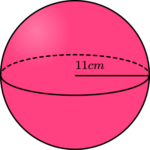(3 marks)

=4\times \pi \times 11^2

(1)

=484\pi=1520.530844…

(1)

1520.53 \; cm^2

(1)

2. Here is a hemisphere with a radius 5 \; mm.(3 marks)

\text{SAH }=3\pi{r}^2=3\times\pi\times{5}^2

(1)

\text{SAH }=75\pi=235.619449…

(1)

236 \; mm^2

(1)

3. A spherical ball has a radius of 30 \; cm.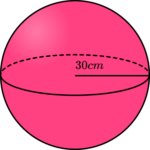A tin of paint covers 2000 \; cm^2.

A tin of paint costs £13.

Calculate how much it would cost to paint the outside surface of the spherical ball.

(3 marks)

4 \times \pi \times 30^2=3600\pi=11309.73355…

(1)

11309.73355… \div {2000} = 5.654866776… = 6 \text{ tins}

(1)

6 \times 13=\pounds{78}

(1)

## Learning checklist

You have now learned how to:

• calculate surface areas of spheres and composite solids

## Did you know?

Archimedes was a famous ancient Greek mathematician who lived about 2,200 years ago in Sicily.  He wrote about the surface area of a sphere and said it was four times the area of the greatest circle.  This is the same formula that we use today.

Archimedes also worked on cylinders and discovered the formula for the lateral surface area of a cylinder.  The lateral surface area excludes its circular base and top.  We would usually refer to this as its curved surface area.

## Still stuck?

Prepare your KS4 students for maths GCSEs success with Third Space Learning. Weekly online one to one GCSE maths revision lessons delivered by expert maths tutors.

Find out more about our GCSE maths tuition programme.